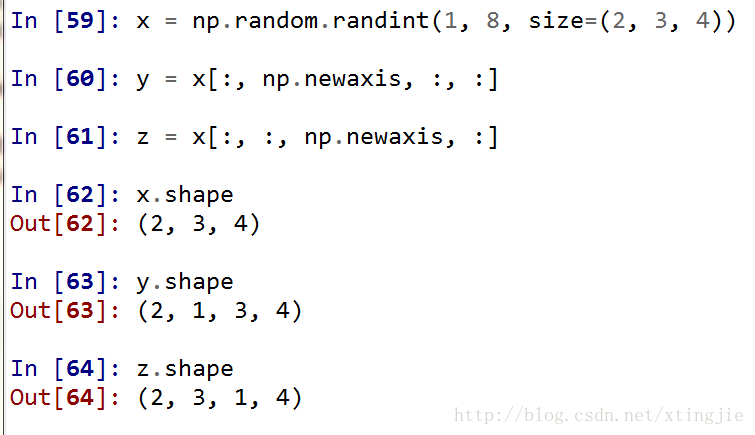# Numpy adds a new dimension: newaxis

Newaxis contained in

numpy can add one dimension to the original array

np.newaxis produces a different array

depending on where it is placed

# one-dimensional array

x = np.random.randint(1, 8, size=5)

x
Out: array([4, 6, 6, 6, 5])

x1 = x[np.newaxis, :]

x1
Out: array([[4, 6, 6, 6, 5]])

x2 = x[:, np.newaxis]

x2
Out:
array([,
,
,
,
])

as you can see from the above code,

when putting newaxis first

, which used to be 5, now becomes 1

x
< script type=”math/tex” id=”MathJax-Element-124″> \times< /script> 5, so the first dimension has changed, the second dimension has changed

and when you put newaxis in the end, the shape of the new array that you output is 5

x
< script type=”math/tex” id=”MathJax-Element-125″> \times< /script> So 1, that’s another dimension that’s less than /p>
So, where you put newaxis, you’ll see an extra dimension in your shape that’s less than /p b>

is as follows:# general problem

is often a problem where you need to take a portion of the data out of the array, that is, take a “slice” or a “strip”

, for example, you need to extract a column

from a two-dimensional array

when you take out the dimension becomes one

if we want to reduce it to two dimensions, we need the above method